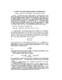## A Note on Conjugate Gradient Convergence##### Files
CS-TR-3516.ps(347.82 KB)
The one-dimensional discrete Poisson equation on a uniform grid with $n$ points produces a linear system of equations with a symmetric positive-definite coefficient matrix. Hence, the conjugate gradient method can be used, and standard analysis gives an upper bound of $O(n)$ on the number of iterations required for convergence. This paper introduces a systematically defined set of solutions dependent on a parameter $\beta$, and for several values of $\beta$, presents exact analytic expressions for the number of steps $k(\beta,\tau,n)$ needed to achieve accuracy $\tau$. The asymptotic behavior of these expressions has the form $O(n^{\alpha})$ as $n \to \infty$ and $O(\tau^{\gamma})$ as $\tau \to \infty$. In particular, two choices of $\beta$ corresponding to nonsmooth solutions give $\alpha=0$, i.e., iteration counts independent of $n$; this is in contrast to the standard bounds. The standard asymptotic convergence behavior, $\alpha=1$, is seen for a relatively smooth solution. Numerical examples illustrate and supplement the analysis. (Also cross-referenced as UMIACS-TR-95-86)